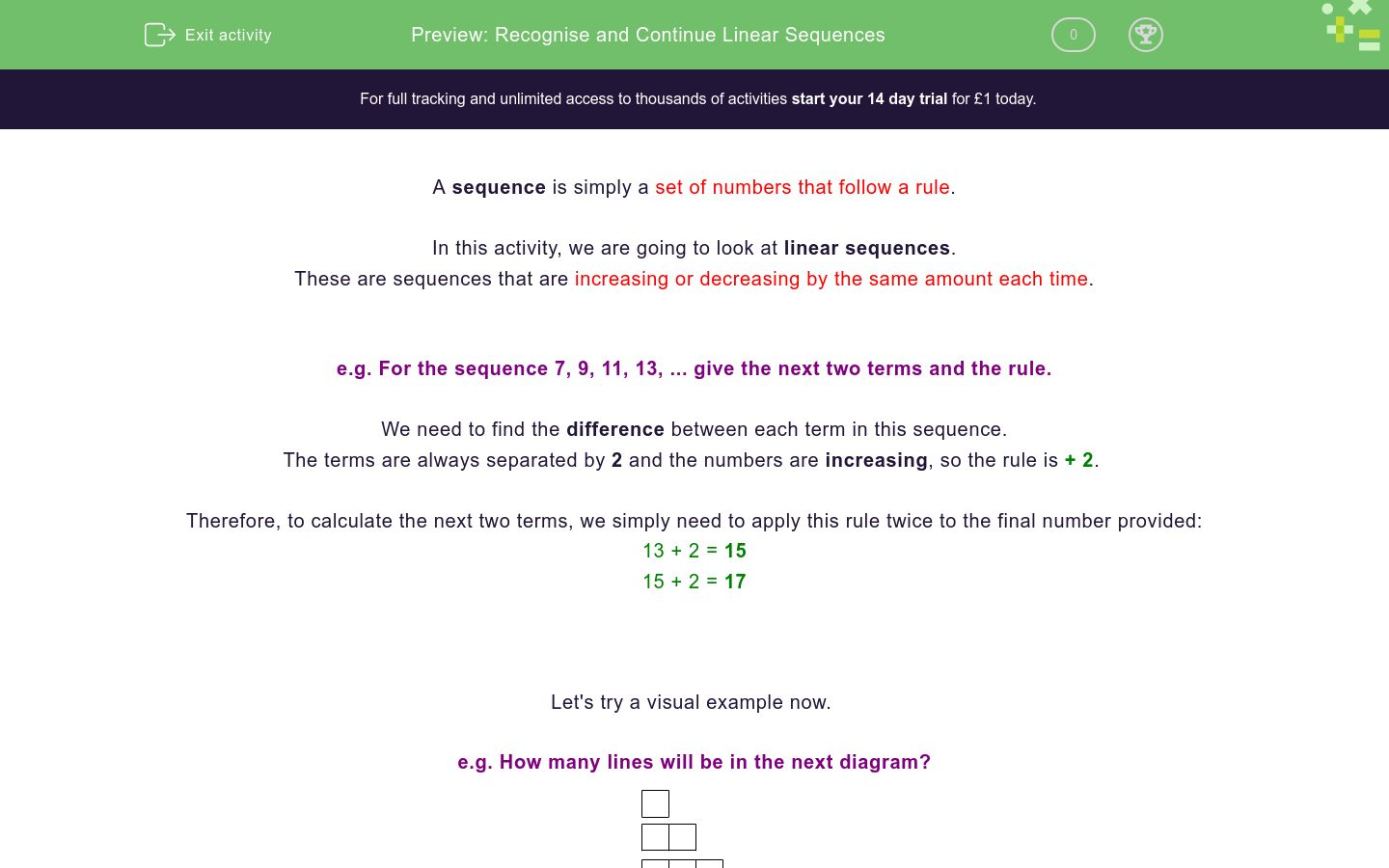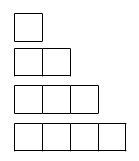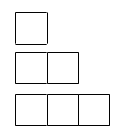# Recognise and Continue Linear Sequences

In this worksheet, students find rules to describe linear sequences (which increase/decrease by the same amount each time), and apply rules to find the next terms in sequences.Key stage:  KS 4

GCSE Subjects:   Maths

GCSE Boards:   AQA, Eduqas, OCR, Pearson Edexcel

Curriculum topic:   Algebra

Curriculum subtopic:   Sequences

Difficulty level:### QUESTION 1 of 10

A sequence is simply a set of numbers that follow a rule.

In this activity, we are going to look at linear sequences.

These are sequences that are increasing or decreasing by the same amount each time.

e.g. For the sequence 7, 9, 11, 13, ... give the next two terms and the rule.

We need to find the difference between each term in this sequence.

The terms are always separated by 2 and the numbers are increasing, so the rule is + 2

Therefore, to calculate the next two terms, we simply need to apply this rule twice to the final number provided:

13 + 2 = 15

15 + 2 = 17

Let's try a visual example now.

e.g. How many lines will be in the next diagram?Whenever we have a question like this, where a sequence is drawn instead of given as numbers, our first step is to always turn it into a sequence which contains numbers.

If we count the lines in each diagram, we reach the following sequence:

4, 7, 10, 13, ...

So the rule is + 3 and the next diagram will have 16 lines.

You might also be asked to draw it, but we're sure you could do that if requested!

In this activity, we will find rules to describe both increasing and decreasing linear sequences (which increase/decrease by the same amount each time), and apply rules to find the next terms in sequences.

In this activity you should not use a calculator, but instead practise your mental calculations.

What are the next two terms in the sequence below?

1, 3, 5, 7, __, __

Match each sequence with its correct rule.

## Column B

1, 4, 7, 10
+ 3
2, 4, 6, 8
- 3
10, 7, 4, 1
+ 0
1, 1, 1, 1
+ 2

Which of the rules in the list below could describe this sequence?

3, 7, 11, 15, ...

× 2

+ 4

- - 4

+ 5

Which of the rules in the list below could describe this sequence?

10, 8, 6, 4, ...

+ 2

- 2

- - 2

- + 2

How many lines will the next diagram in the sequence below have?How many lines will the sixth diagram in the sequence below have?For each sequence and accompanying rule below, check the box to describe if the relationship shown is correct or incorrect

True or false?

A sequence can only increase or decrease by the same amount each time.

True

False

What are the next three terms in this sequence?

25, 22, 19, __, __, __

True

False

Complete the sentence below by adding the correct missing word into the gap.

True

False

• Question 1

What are the next two terms in the sequence below?

1, 3, 5, 7, __, __

EDDIE SAYS
Did you look for the difference between the terms to find the rule? We have to add 2 each time. So let's add 2 to 7 to find the first missing term, then add 2 to this answer to find the next: 7 + 2 = 9 9 + 2 = 11 How did you find this first challenge?
• Question 2

Match each sequence with its correct rule.

## Column B

1, 4, 7, 10
+ 3
2, 4, 6, 8
+ 2
10, 7, 4, 1
- 3
1, 1, 1, 1
+ 0
EDDIE SAYS
Just remember that we need to find the differences between each of the terms in each sequence, one at a time. If the numbers are increasing, then the rule will use a + sign, but if they are decreasing it will use a - sign. Let's look at the first option as an example: 1, 4, 7, 10 The difference between each term is 3 and the sequence is increasing, therefore our rule is + 3. Can you follow this example to find the remaining rules independently?
• Question 3

Which of the rules in the list below could describe this sequence?

3, 7, 11, 15, ...

+ 4
- - 4
EDDIE SAYS
You probably spotted one of the rules quite easily, but did you notice the other? This sequence has a difference of 4 between the terms and is increasing, so the simplest way to express this rule is + 4. However, remember that when two minus symbols are placed next to each other, they become a plus. So - - 4 also means the same thing. Well done if you spotted that!
• Question 4

Which of the rules in the list below could describe this sequence?

10, 8, 6, 4, ...

- 2
- + 2
EDDIE SAYS
Did you consider the relationships between - and + here? Our sequence here is decreasing and the difference between the terms is 2, so the simplest rule to use is - 2. However, as a - next to a + also makes a - overall, - + 2 also means the same thing.
• Question 5

How many lines will the next diagram in the sequence below have?9
EDDIE SAYS
Let's start by turning this visual into a number sequence. If we count the lines in each image, we get: 3, 5, 7, ... We can see here that our sequence is increasing and the difference between the terms is 2, so our rule to apply is + 2. 7 + 2 = 9 Could you draw this image if you were asked to?
• Question 6

How many lines will the sixth diagram in the sequence below have?19
EDDIE SAYS
The common mistake here is to count the number of lines in the third diagram and double it to find the sixth. Unfortunately, it's not quite that simple. We need to find the rule for the sequence and then apply it to reach the sixth term. Let's count the lines to start, our sequence here is: 4, 7, 10, ... We can see that it is increasing by 3 each time, so our rule to apply is + 3. 4th: 10 + 3 = 13 5th: 13 + 3 = 16 6th: 16 + 3 = 19 Could you draw the sixth diagram in this sequence if requested to?
• Question 7

For each sequence and accompanying rule below, check the box to describe if the relationship shown is correct or incorrect

EDDIE SAYS
Take each sequence one at a time, calculate the difference and notice if it increasing or decreasing. Let's look at the first sequence together. It is increasing by 1 each time, so the rule of + 1 is correct here. It's really easy to make a mistake if you're rushing, so be sure to take your time.
• Question 8

True or false?

A sequence can only increase or decrease by the same amount each time.

False
EDDIE SAYS
We have only dealt with sequences that do increase or decrease by the same amount each time, but this doesn't mean that nothing else could possibly happen. Think about the sequence: 2, 4, 8, 16, ... Doesn't this have a rule too? The sequences we have been focusing on, which do increase or decrease by the exact same amount each time, are a special type of sequence, known as linear sequences.
• Question 9

What are the next three terms in this sequence?

25, 22, 19, __, __, __

EDDIE SAYS
Each term in this sequence is decreasing by 3 each time. So to find the next three terms we need to perform the following calculations: 4th: 19 - 3 = 16 5th: 16 - 3 = 13 6th: 13 - 3 = 10
• Question 10

Complete the sentence below by adding the correct missing word into the gap.

EDDIE SAYS
We need to make sure we use the correct vocabulary when working with sequences. The correct way to say 'look at how much it goes up each time' is to 'find the difference'. This is an important term to know, use and be on the lookout for in questions. Great job completing this activity on linear sequences! If you are ready for a challenge, why not try a Level 2 activity focusing on this same topic?
---- OR ----

Sign up for a £1 trial so you can track and measure your child's progress on this activity.

### What is EdPlace?

We're your National Curriculum aligned online education content provider helping each child succeed in English, maths and science from year 1 to GCSE. With an EdPlace account you’ll be able to track and measure progress, helping each child achieve their best. We build confidence and attainment by personalising each child’s learning at a level that suits them.

Get started